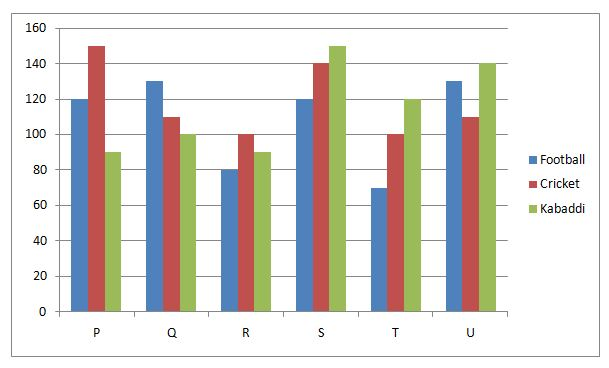# IBPS SO / NIACL AO Prelims – Quantitative Aptitude Questions Day- 56

Dear Readers, Bank Exam Race for the Year 2018 is already started, To enrich your preparation here we have providing new series of Practice Questions on Quantitative Aptitude – Section. Candidates those who are preparing for IBPS SO / NIACL AO Prelims 2018 Exams can practice these questions daily and make your preparation effective.

[WpProQuiz 4634]

Directions (Q. 1 – 5): What value should come in the place of question mark (?) in the following questions?

1) (754 + 432 + 556 + 818) ÷ (21 + 33 – ? + 40) = 32

a) 22

b) 30

c) 14

d) 36

e) None of these

2) 38 % of 350 + 400 % of 16 =? % of 50

a) 394

b) 416

c) 358

d) 312

e) None of these

3) (1560 ÷ 40) + (4/7) of (5/8) of 56 = 24 +?

a) 49

b) 42

c) 28

d) 35

e) None of these

4) √x × 120 = (23481 – 22761) × 11

a) 5325

b) 4356

c) 4185

d) 7395

e) None of these

5) (8/3) of [908 + 1279] + 38 =? + 350

a) 3160

b) 3580

c) 5520

d) 4850

e) None of these

Directions (Q. 6 – 10) Study the following information carefully and answer the given questions:

The following bar graph shows the total number of students playing different games from various schools.6) Find the difference between the total students playing all the given games together in school P to that of school R?

a) 90

b) 110

c) 130

d) 75

e) None of these

7) Find the ratio between the total number of students playing Football in School Q, R and S together to that of total number of students playing Kabaddi in School P, T and U together?

a) 12: 17

b) 21: 29

c) 15: 23

d) 33: 35

e) None of these

8) Find the average number of students playing Cricket in all the given schools together except U?

a) 135

b) 120

c) 125

d) 115

e) None of these

9) The total number of students playing all the given games together in school S is approximately what percentage more than the total number of students playing all the given games together in school R?

a) 52 %

b) 66 %

c) 44 %

d) 38 %

e) 26 %

10) Total number of students playing football in all the given schools together is approximately what percentage of total number of students playing Cricket in all the given schools together?

a) 106 %

b) 124 %

c) 92 %

d) 135 %

e) 80 %

Directions (Q. 1 – 5):

(754 + 432 + 556 + 818) ÷ (21 + 33 – x + 40) = 32

2560/(94 – x) = 32

2560/32 = 94 – x

80 = 94 – x

X = 94 – 80 = 14

38 % of 350 + 400 % of 16 = x % of 50

(38/100)*350 + (400/100)*16 = (x/100)*50

133 + 64 = x/2

197*2 = x

X = 394

(1560 ÷ 40) + (4/7) of (5/8) of 56 = 24 + x

(1560/40) + (4/7)*(5/8)*56 = 24 + x

39 + 20 – 24 = x

X = 35

√x × 120 = (23481 – 22761) × 11

√x × 120 = 720*11

√x = (720*11)/120 = 66

= > x = 4356

(8/3) of [908 + 1279] + 38 = x + 350

(8/3)*(2187) + 38 = x + 350

X = 5832 + 38 – 350 = 5520

Directions (Q. 6 -10):

The total students playing all the given games together in school P

= > 120 + 150 + 90 = 360

The total students playing all the given games together in school R

= > 80 + 100 + 90 = 270

Required difference = 360 – 270 = 90

The total number of students playing Football in School Q, R and S together

= > 130 + 80 + 120 = 330

The total number of students playing Kabaddi in School P, T and U together

= > 90 + 120 + 140 = 350

Required ratio = 330: 350 = 33: 35

The total number of students playing Cricket in all the given schools together except U

= > 150 + 110 + 100 + 140 + 100 = 600

Required average = 600/5 = 120

The total number of students playing all the given games together in school S

= > 120 + 140 + 150 = 410

The total number of students playing all the given games together in school R

= > 80 + 100 + 90 = 270

Required % = [(410 – 270)/270]*100 = 52 %

Total number of students playing football in all the given schools together

= > 120 + 130 + 80 + 120 + 70 + 130 = 650

Total number of students playing Cricket in all the given schools together

= > 150 + 110 + 100 + 140 + 100 + 110 = 710

Required % = (650/710)*100 = 92 %

Daily Practice Test Schedule | Good Luck

 Topic Daily Publishing Time Daily News Papers & Editorials 8.00 AM Current Affairs Quiz 9.00 AM Current Affairs Quiz (Hindi) 9.30 AM IBPS SO/NIACL AO Prelims – Reasoning 10.00 AM IBPS SO/NIACL AO Prelims – Reasoning (Hindi) 10.30 AM IBPS SO/NIACL AO Prelims – Quantitative Aptitude 11.00 AM IBPS SO/NIACL AO Prelims – Quantitative Aptitude (Hindi) 11.30 AM Vocabulary (Based on The Hindu) 12.00 PM IBPS SO/NIACL AO Prelims – English Language 1.00 PM SSC Practice Questions (Reasoning/Quantitative aptitude) 2.00 PM IBPS Clerk – GK Questions 3.00 PM SSC Practice Questions (English/General Knowledge) 4.00 PM Daily Current Affairs Updates 5.00 PM SBI PO/IBPS Clerk Mains – Reasoning 6.00 PM SBI PO/IBPS Clerk Mains – Quantitative Aptitude 7.00 PM SBI PO/IBPS Clerk Mains – English Language 8.00 PM